A thermodynamic system is taken from state A to B along ACB and is brought back to A along BDA as shown in the PV diagram. The net work done during the complete cycle is given by the area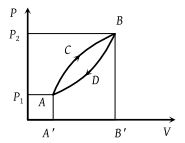(1) P1ACBP2P1

(2) ACBB'A'A

(3) ACBDA

Concept Questions :-

Work done by gas
High Yielding Test Series + Question Bank - NEET 2020

Difficulty Level:

In the diagrams (i) to (iv) , variation of volume with changing pressure is shown. A gas is taken along the path ABCD. The change in internal energy of the gas will be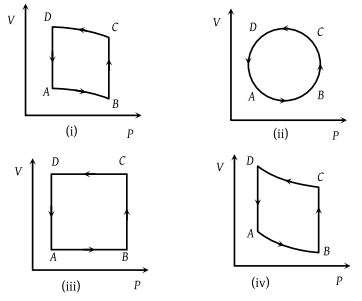(1) Positive in all cases (i) to (iv)

(2) Positive in cases (i), (ii) and (iii) but zero in (iv) case

(3) Negative in cases (i), (ii) and (iii) but zero in (iv) case

(4) Zero in all four cases

Concept Questions :-

Cyclic process
High Yielding Test Series + Question Bank - NEET 2020

Difficulty Level:

The P-V graph of an ideal gas cycle is shown here as below. The adiabatic process is described by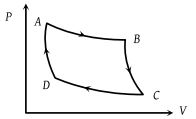(1) AB and BC

(2) AB and CD

(3) BC and DA

(4) BC and CD

Concept Questions :-

Types of processes
High Yielding Test Series + Question Bank - NEET 2020

Difficulty Level:

An ideal monoatomic gas is taken round the cycle  as shown in following P-V diagram. The work done during the cycle is -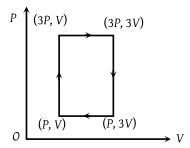(1) PV

(2) 2 PV

(3) 4 PV

(4) Zero

Concept Questions :-

Work done by gas
High Yielding Test Series + Question Bank - NEET 2020

Difficulty Level:

A system changes from the state (P1, V1) to (P2, V2) as shown in the figure. What is the work done by the system ?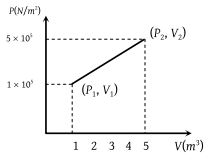(1) 7.5 × 105 joule

(2) 7.5 × 105 erg

(3) 12 × 105 joule

(4) 6 × 105 joule

Concept Questions :-

Work done by gas
High Yielding Test Series + Question Bank - NEET 2020

Difficulty Level:

Carbon monoxide is carried around a closed cycle abc in which bc is an isothermal process as shown in the figure. The gas absorbs 7000 J of heat as its temperature increases from 300 K to 1000 K in going from a to b. The quantity of heat rejected by the gas during the process ca is -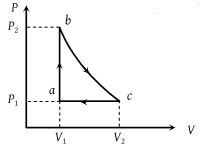(1) 4200 J

(2) 5000 J

(3) 9000 J

(4) 9800 J

Concept Questions :-

Cyclic process
High Yielding Test Series + Question Bank - NEET 2020

Difficulty Level:

A sample of ideal monoatomic gas is taken round the cycle CBAC as shown in the figure. The work done during the cycle is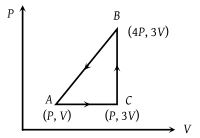(1) Zero

(2) 3 PV

(3) 6 PV

(4) 9PV

Concept Questions :-

Work done by gas
High Yielding Test Series + Question Bank - NEET 2020

Difficulty Level:

When a system is taken from state i to a state f along path iaf, Q = 50 J and W = 20 J. Along path ibf, Q = 35 J. If W = –13 J for the curved return path f i, Q for this path is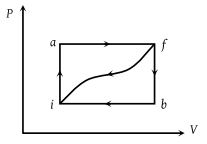(1) 33 J

(2) 23 J

(3) – 7 J

(4) – 43 J

Concept Questions :-

First law of thermodynamics
High Yielding Test Series + Question Bank - NEET 2020

Difficulty Level:

For one complete cycle of a thermodynamic process on a gas as shown in the P-V diagram, which of following is correct ?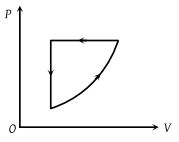(1) $\Delta {E}_{\mathrm{int}}=0,\text{\hspace{0.17em}}Q<0$

(2) $\Delta {E}_{\mathrm{int}}=0,\text{\hspace{0.17em}}Q>0$

(3) $\Delta \text{\hspace{0.17em}}{E}_{\mathrm{int}}>0,\text{\hspace{0.17em}}Q<0$

(4) $\Delta \text{\hspace{0.17em}}{E}_{\mathrm{int}}<0,\text{\hspace{0.17em}}Q>\text{​\hspace{0.17em}}0$

Concept Questions :-

Cyclic process
High Yielding Test Series + Question Bank - NEET 2020

Difficulty Level:

An ideal gas is taken from point A to the point B, as shown in the P-V diagram. The work done in the process is -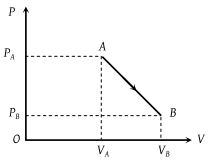(1) $\left({P}_{A}-{P}_{B}\right)\left({V}_{B}-{V}_{A}\right)$

(2) $\frac{1}{2}\left({P}_{B}-{P}_{A}\right)\left({V}_{B}+{V}_{A}\right)$

(3) $\frac{1}{2}\left({P}_{B}-{P}_{A}\right)\left({V}_{B}-{V}_{A}\right)$

(4) $\frac{1}{2}\left({P}_{B}+{P}_{A}\right)\left({V}_{B}-{V}_{A}\right)$

Concept Questions :-

Work done by gas